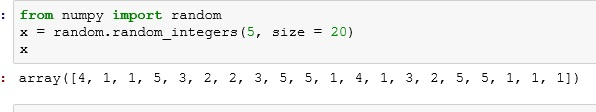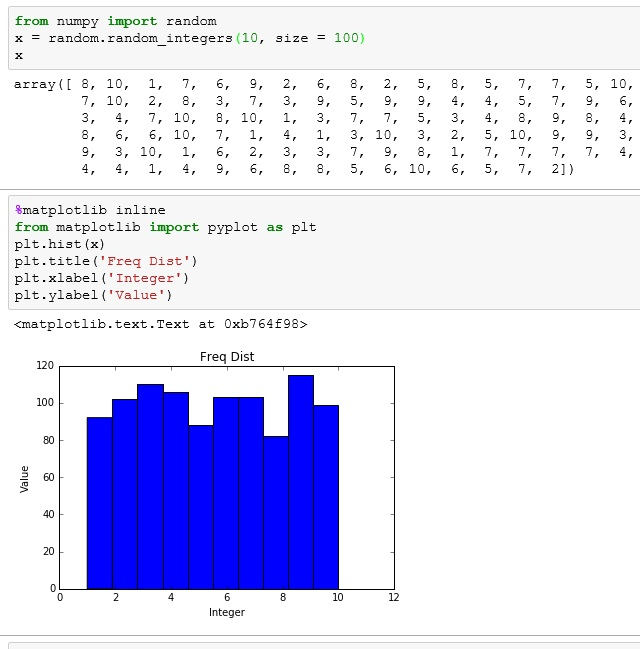# Python: Histograms and Frequency Distribution

In the spirit total transparency, this is a lesson is a stepping stone towards explaining the Central Limit Theorem. While I promise not to bog this website down with too much math, a basic understanding of this very important principle of probability is an absolute need.

## Frequency Distribution

To understand the Central Limit Theorem, first you need to be familiar with the concept of Frequency Distribution.

Let’s look at this Python code below. Here I am importing the module random from numpy. I then use the function random_integers from random. Here is the syntax:

random.random_integers(Max value, number of elements)

So random.random_integers(10, size =10) would produce a list of 10 numbers between 1 and 10.

Below I selected 20 numbers between 1 and 5Now, since I am talking about a Frequency Distribution, I’d bet you could infer that I am concerned with Frequency. And you would be right. Looking at the data above, this is what I have found.

I create a table of the integers 1 – 5 and I then count the number of time (frequency) each number appears in my list above.## Histogram

Using my Frequency table above, I can easily make a bar graph commonly known as a histogram. However, since this is a Python lesson as well as a Probability lesson, let’s use matplotlab to build this.

The syntax should be pretty self explanatory if you have viewed my earlier Python graphing lessons.Now lets, do it with even more data points (100 elements from 1 to 10 to be exact)If you enjoyed this lesson, click LIKE below, or even better, leave me a COMMENT.

1.Anonymous
1.Marshall Gu